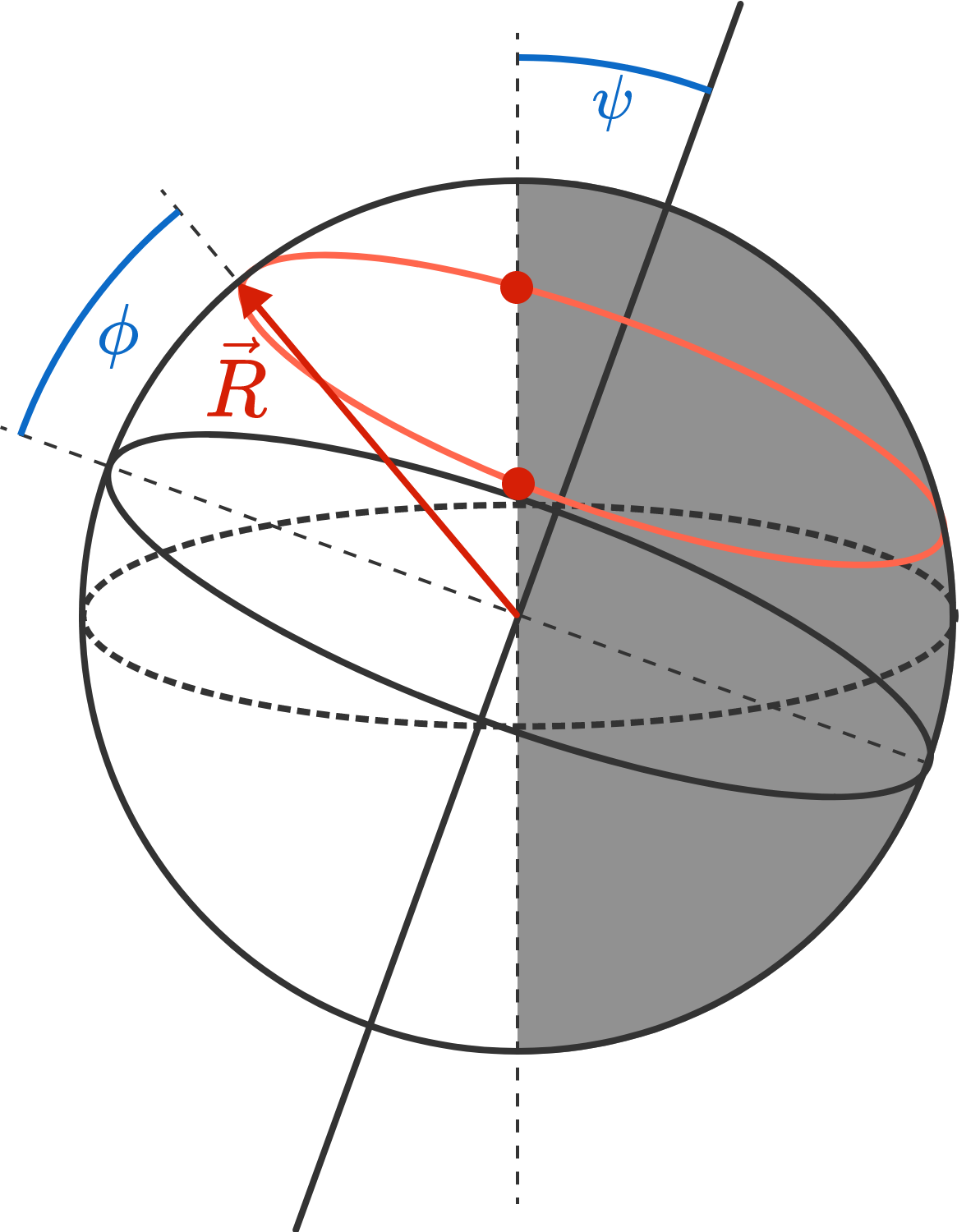# Winter solstice

Geometry Level 2Approximately how long is the daytime (the time between sunrise and sunset) on the $21^\text{st}$ of December in Paris?

Note: Paris is on the latitude $\phi = 49^\circ.$ The Earth is tilted at an angle of $\psi = 23.4^\circ$ relative to its axis of rotation.

×